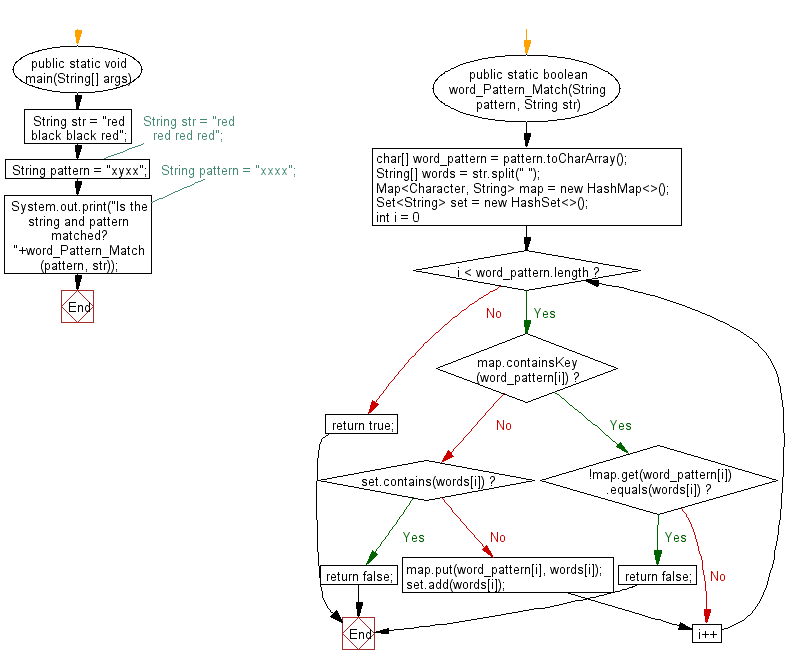# Java Exercises: Check a string follows a specified pattern

## Java Basic: Exercise-199 with Solution

Write a Java program to check a string follows a given pattern.

Example pattern:
Given pattern = "xyyx", str = "red black black red", return true.
Given pattern = "xyyx", str = "red black black green", return false.
Given pattern = "xxxx", str = "red black black red", return false.
Given pattern = "xxxx", str = "red red red red", return true.

Sample Solution:

Java Code:

``````import java.util.*;
public class Solution {
public static void main(String[] args) {
String str = "red black black red";
//String str = "red red red red";
String pattern = "xyxx";
//String pattern = "xxxx";
System.out.print("Is the string and pattern matched? " + word_Pattern_Match(pattern, str));
}

public static boolean word_Pattern_Match(String pattern, String str) {
char[] word_pattern = pattern.toCharArray();
String[] words = str.split(" ");

Map < Character, String > map = new HashMap < > ();
Set < String > set = new HashSet < > ();

for (int i = 0; i < word_pattern.length; i++) {
if (map.containsKey(word_pattern[i])) {
if (!map.get(word_pattern[i]).equals(words[i])) {
return false;
}
continue;
}

if (set.contains(words[i])) {
return false;
}
map.put(word_pattern[i], words[i]);
}
return true;
}
}
```
```

Sample Output:

```Is the string and pattern matched? false
```

Flowchart:Java Code Editor:

Company: